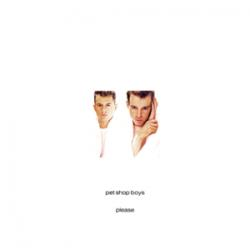Two Divided By Zero

Pet Shop Boys

LETRA

Two Divided By Zero Letra

(Two divided by zero, zero)
(Two divided by zero, zero, zero)

Let's not go home, we'll catch the late train
I've got enough money to pay all the way
When the postman calls, he'll deliver the letter
I've explained everything; it's better that way

(Divided by, divided by) I think they heard a rumor
(Divided by, divided by) Or someone tipped them off
(Divided by, divided by) It's better to go sooner
(Divided by, divided by) Than call it all off

We'll catch a plane to New York, and a cab going down
Cross the bridges and tunnels, straight into town
Tomorrow morning we'll be miles away
On another continent and another day

(Divided by, divided by) Let's not go home
(Divided by, divided by) Or call it a day
(Divided by, divided by) You won't be alone
(Divided by, divided by) Let's run away

(Two divided by zero, zero)
(Two divided by zero, zero, zero)
(D, d, d, d, d, divided by)
(Divided by, divided by)
(Z, z, z, z, z, zero, zero)
(Divided by)

(Turn of that noise)

(Divided by, divided by) Someone spread a rumor
(Divided by, divided by zero, zero)
(Divided by, divided by) Better to go sooner
(Divided by, divided by) Let's run away

So why hang around for the deed to be done
You can give it all up for a place in the sun
When the postman calls we'll be miles away
On a plane to New York and another day

(Divided by, divided by) I think they heard a rumor
(Divided by, divided by) Or someone tipped them off
(Divided by, divided by) Better to go sooner
(Divided by, divided by) Than call it all off

(Divided by, divided by) Someone spread a rumor
(Divided by, divided by) And someone has to pay
(Divided by, divided by) Let's not go home
(Divided by, divided by) Let's run away
(Divided by, divided by) Let's not go home
(Divided by, divided by) Let's run away